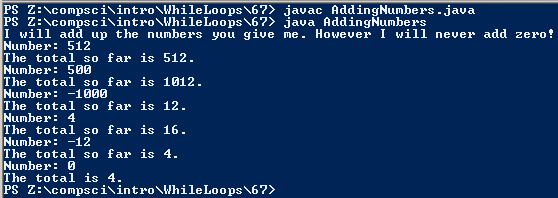# Assignemnt #67 and Adding Numbers

## Code

```    ///Name: Daniel Tiffany-Appleton
///Period: 7
///Date Finished: 12/3/15

import java.util.Scanner;

{
public static void main( String [] args )
{

Scanner keyboard = new Scanner(System.in);

int userNumber, amount, total;

System.out.println( "I will add up the numbers you give me. However I will never add zero!");
System.out.print( "Number: ");
userNumber = keyboard.nextInt();
total = userNumber;

while( userNumber != 0 )
{

System.out.println( "The total so far is " + total + "." );
amount = total;
System.out.print( "Number: ");
userNumber = keyboard.nextInt();
total = amount + userNumber;

}

System.out.println( "The total is " + total + "." );

}
}
```

### Picture of the output